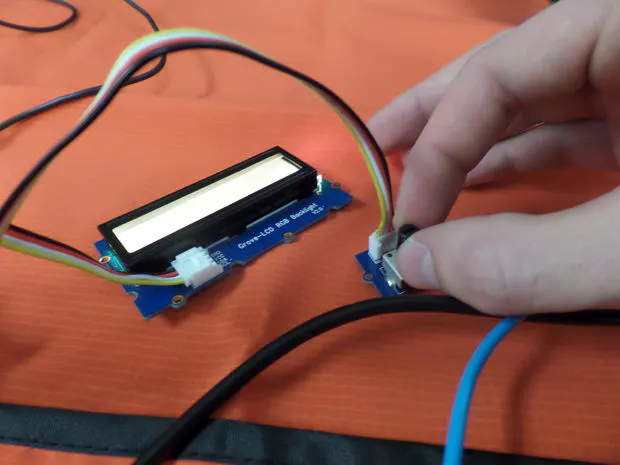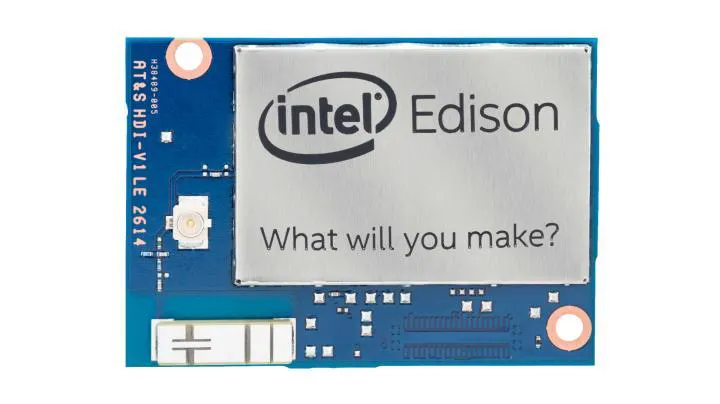Published

# COI - Party Lighting

Control fancy lights with an Intel Edison or Arduino!

Full instructions provided588## Things used in this project

### Hardware componentsIntel Edison
×1
 Static Mat
×1
 Mini USB Cables
×2
 Jumper Cables
×2
 LEDs 1 blue, 1 green, and 1 red
×3
 1K ohm resistors
×3×1
 4-pin cables
×1
 Seeed RGB Backlight LCD
×1
 Seeed Rotary Angle Sensor
×1
 Computer
×1

## Code

### Code

C/C++
```void loop() {
lcd.setCursor(0,0);
//lcd.setCursor(0,1);
double fraction = (read - minPotValue)/(maxPotValue - minPotValue);
fraction = fraction - floor(fraction);
//lcd.print(fraction);
double sixth = 1.0/6.0;
int r = 0;
int g = 0;
int b = 0;
if(fraction < sixth){
r = 255;
g = (int)((fraction)/sixth * 255);
} else if(fraction < 2 * sixth){
r = (int)((2 * sixth - fraction)/sixth * 255);
g = 255;
} else if(fraction < 3 * sixth){
g = 255;
b = (int)((fraction-2*sixth)/sixth * 255);
} else if(fraction < 4 * sixth){
g = (int)((4 * sixth - fraction)/sixth * 255);
b = 255;
} else if(fraction < 5 * sixth){
b = 255;
r = (int)((fraction-4*sixth)/sixth * 255);
} else {
r = 255;
b = (int)((6 * sixth - fraction)/sixth * 255);
}
analogWrite(redPinNum, 255 - r); //because of inverted values
analogWrite(greenPinNum, 255-g);
analogWrite(bluePinNum, 255-b);
lcd.setRGB(r,g,b);
}
```